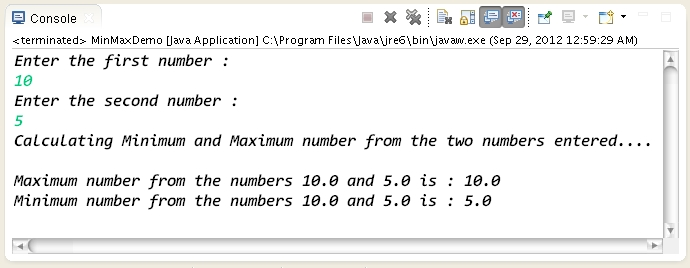How to calculate minimum and maximum of a number using Math class in Java ?.

 Tweet Program to demonstrate how to calculate minimum and maximum of a number using Math class in Java package com.hubberspot.code; import java.util.Scanner; public class MinMaxDemo { public static void main(String[] args) { // In order to calculate minimum and maximum of // number from the two numbers we use max and min // method of Math class // Create a Scanner object which takes System.in object // this makes us read values from the console // We will ask user to input two numbers Scanner scanner = new Scanner(System.in); // Prompting user to enter first number ... System.out.println("Enter the first number : "); double number1 = 0; // Scanner objects nextDouble reads output from the console // entered by user and stores into the double variable number1 = scanner.nextDouble(); // Prompting user to enter second number ... System.out.println("Enter the second number : "); double number2 = 0; number2 = scanner.nextDouble(); System.out.println("Calculating Minimum and Maximum number " + "from the two numbers entered.... "); System.out.println(); // Math.max method calculates the maximum number from the // two numbers passed to it as a argument // Math.min method calculates the minimum number from the // two numbers passed to it as a argument double maximum = Math.max(number1, number2); double minimum = Math.min(number1, number2); System.out.println("Maximum number from the numbers " + number1 + " and " + number2 + " is : " + maximum); System.out.println("Minimum number from the numbers " + number1 + " and " + number2 + " is : " + minimum); } } Output of the program :Program to demonstrate how to calculate minimum and maximum of a number using Math class in Java

package com.hubberspot.code;

import java.util.Scanner;

public class MinMaxDemo {

public static void main(String[] args) {

// In order to calculate minimum and maximum of
// number from the two numbers we use max and min
// method of Math class

// Create a Scanner object which takes System.in object
// this makes us read values from the console
// We will ask user to input two numbers
Scanner scanner = new Scanner(System.in);

// Prompting user to enter first number ...
System.out.println("Enter the first number : ");
double number1 = 0;
// Scanner objects nextDouble reads output from the console
// entered by user and stores into the double variable
number1 = scanner.nextDouble();

// Prompting user to enter second number ...
System.out.println("Enter the second number : ");
double number2 = 0;
number2 = scanner.nextDouble();

System.out.println("Calculating Minimum and Maximum number " +
"from the two numbers entered.... ");

System.out.println();

// Math.max method calculates the maximum number from the
// two numbers passed to it as a argument
// Math.min method calculates the minimum number from the
// two numbers passed to it as a argument

double maximum = Math.max(number1, number2);
double minimum = Math.min(number1, number2);

System.out.println("Maximum number from the numbers "
+ number1 + " and " + number2 + " is : " + maximum);
System.out.println("Minimum number from the numbers "
+ number1 + " and " + number2 + " is : " + minimum);

}

}

Output of the program :

Please Give Us Your 1 Minute In Sharing This Post!
SOCIALIZE IT →